# Preface

This section provides the general introduction to vector theory including inner and outer products. It also serves as a tutorial to operate with vectors using Mathematica. Although vectors have physical meaning in real life, they can be uniquely identified with an ordered tuple of real (or complex numbers). The latter is heavily used in computers to store data as arrays or lists.

Introduction to Linear Algebra with Mathematica

Example 1:    ■

Theorem 1:
---->

# How to define vectors

A vector is a quantity that has both magnitude and direction. Recall that in contrast to a vector, a scalar has only a magnitude. It is commonly represented by a directed line segment whose length is the magnitude and with an arrow indicating the direction in space: $$\overleftarrow{v}$$ or $$\overrightarrow{v} .$$ However, we denote vectors using boldface as in a. The magnitude of a vector is called the norm or length, and it is denoted by double vertical lines, as ∥a∥. The direction of the vector is from its tail to its head. Two geometric vectors are equal if they have the same magnitude and direction. This means that we are allowed to translate a vector to a new location (without rotating it); for instance, starting at the origin.

The main reason why vectors are so useful and popular is that we can do operations with them similarly to ordinary algebra. Namely, there is an internal operation on vectors called addition together with its negation---subtraction. So two vectors can be added or subtracted. Besides these two internal arithmetic operations, there is another outer operation that admits multiplication of a vector by a scalar (real or complex numbers). It is also assumed that there exists a unique zero vector (of zero magnitude and no direction), which can be added/subtracted from any vector without changing the outcome. The zero vector is not the number zero, but it is obtained upon multiplication of any vector by scalar zero. When discussing vectors geometrically, we assume that scalars are real numbers.

• a + b = b + a   (commutative law);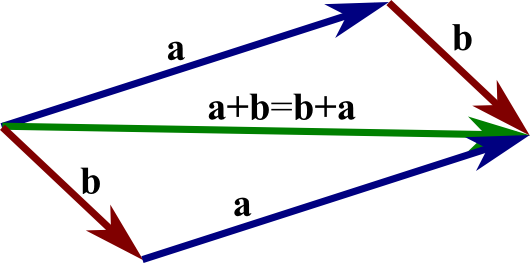Commutative law
• (a + b) + c = a + (b + c)   (associative law);
• There is a vector 0 such that b + 0 = b (additive identity);
• For any vector a, there is a vector −a such that a + (−a) = 0   (Additive inverse).

# Scalar multiplication

Given a vector a and a real number (scalar) λ, we can form the vector λa as follows. If λ is positive, then λa is the vector whose direction is the same as the direction of a and whose length is λ times the length of a. In this case, multiplication by λ simply stretches (if λ>1) or compresses (if 0<λ<1) the vector a. If, on the other hand, λ is negative, then we have to take the opposite of a before stretching or compressing it. In other words, the vector λa points in the opposite direction of a, and the length of λa is |λ| times the length of a. No matter the sign of λ, we observe that the magnitude of λa is |λ| times the magnitude of a: ∥λa∥ = |λ| ∥a∥. Scalar multiplications satisfies many of the same properties as the usual multiplication.
• λ(a + b) = λa + λb   (distributive law, for vectors)
• (λ + β)a = λa + βb   (distributive law for scalars);
• a = a;
• (−1)·a = −a;
• a = 0.
In the last formula, the zero on the left is the scalar 0, while the zero on the right is the vector 0, which is the unique vector whose length is zero.
If a = λb for some scalar λ, then we say that the vectors a and b are parallel. If λ is negative, then it is a common slang to say that a and b are anti-parallel, but we will not use that language.

Generalizing well-known examples of vectors (velocity and force) in physics and engineering, mathematicians introduced abstract object called vectors. So vectors are objects that can be added/subtracted and multiplied by scalars. These two operations (internal addition and external scalar multiplication) are assumed to satisfy natural conditions described above. A set of vectors is said to form a vector space (also called a linear space), if any vectors from it can be added/subtracted and multiplied by scalars, subject to regular properties of addition and multiplication. Wind, for example, has both a speed and a direction and, hence, is conveniently expressed as a vector. The same can be said of moving objects, momentum, forces, electromagnetic fields, and weight. (Weight is the force produced by the acceleration of gravity acting on a mass.)

The first thing we need to know is how to define a vector so it will be clear to everyone. Today more than ever, information technologies are an integral part of our everyday lives. That is why we need a tool to model vectors on computers. One of the common ways to do this is to introduce a system of coordinates, either Cartesian or any other. In engineering, we traditionally use the Cartesian coordinate system that specifies any point with a string of digits. Each coordinate measures a distance from a point to its perpendicular projections onto the mutually perpendicular hyperplanes.

Let us start with our familiar three dimensional space in which the Cartesian coordinate system consists of an ordered triplet of lines (the axes) that go through a common point (the origin), and are pair-wise perpendicular; it also includes an orientation for each axis and a single unit of length for all three axes. Every point is assigned distances to three mutually perpendicular planes, called coordinate planes (such that the pair x and y axes define the z-plane, x and z axes define the y-plane, etc.). The reverse construction determines the point given its three coordinates. Each pair of axes defines a coordinate plane. These planes divide space into eight trihedra, called octants. The coordinates are usually written as three numbers (or algebraic formulas) surrounded by parentheses and separated by commas, as in (-2.1,0.5,7). Thus, the origin has coordinates (0,0,0), and the unit points on the three axes are (1,0,0), (0,1,0), and (0,0,1).

There are no universal names for the coordinates in the three axes. However, the horizontal axis is traditionally called abscissa borrowed from New Latin (short for linear abscissa, literally, "cut-off line"), and usually denoted by x. The next axis is called ordinate, which came from New Latin (linea), literally, line applied in an orderly manner; we will usually label it by y. The last axis is called applicate and usually denoted by z. Correspondingly, the unit vectors are denoted by i (abscissa), j (ordinate), and k (applicate), called the basis. Once rectangular coordinates are set up, any vector can be expanded through these unit vectors. In the three dimensional case, every vector can be expanded as $${\bf v} = v_1 {\bf i} + v_2 {\bf j} + v_3 {\bf k} ,$$ where $$v_1, v_2 , v_3$$ are called the coordinates of the vector v. Coordinates are always specified relative to an ordered basis. When a basis has been chosen, a vector can be expanded with respect to the basis vectors and it can be identified with an ordered n-tuple of n real (or complex) numbers or coordinates. The set of all real (or complex) ordered numbers is denoted by ℝn (or ℂn). In general, a vector in infinite dimensional space is identified by an infinite sequence of numbers. Finite dimensional coordinate vectors can be represented by either a column vector (which is usually the case) or a row vector. We will denote column-vectors by lower case letters in bold font, and row-vectors by lower case letters with a superimposed arrow. Because of the way the Wolfram Language uses lists to represent vectors, Mathematica does not distinguish column vectors from row vectors, unless the user specifies which one is defined. One can define vectors using Mathematica commands: List, Table, Array, or curly brackets.

In mathematics and applications, it is a custom to distinguish column vectors

${\bf v} = \left( \begin{array}{c} v_1 \\ v_2 \\ \vdots \\ v_m \end{array} \right) \qquad \mbox{also written as } \qquad {\bf v} = \left[ \begin{array}{c} v_1 \\ v_2 \\ \vdots \\ v_m \end{array} \right] ,$
for which we use lowercase letters in boldface type, from row vectors (ordered n-tuple)
$\vec{v} = \left[ v_1 , v_2 , \ldots , v_n \right] .$
Here entries $$v_i$$ are known as the component of the vector. The column vectors and the row vectors can be defined using matrix command as an example of an $$n\times 1$$ matrix and $$1\times n$$ matrix, respectively.

The concept of a vector space (also a linear space) has been defined abstractly in mathematics. Historically, the first ideas leading to vector spaces can be traced back as far as the 17th century; however, the idea crystallized with the work of the German mathematician Hermann Günther Grassmann (1809--1877), who published a paper in 1862. A vector space is a collection of objects called vectors, which may be added together and multiplied ("scaled") by numbers, called scalars, the result producing more vectors in this collection. Scalars are often taken to be real numbers, but there are also vector spaces with scalar multiplication by complex numbers, rational numbers, or generally scalars in any field. The operations of vector addition and scalar multiplication must satisfy certain requirements, called axioms (they can be found on the web page).

Vectors in Mathematica are built, manipulated and accessed similarly to matrices (see next section). However, as simple lists (“one-dimensional,” not “two-dimensional” such as matrices that look more tabular), they are easier to construct and manipulate. They will be enclosed in brackets ( [,] ) which allows us to distinguish a vector from a matrix with just one row, if we look carefully. The number of “slots” in a vector is not referred to in Mathematica as rows or columns, but rather by “size.”

In Mathematica, defining vectors and matrices is done by typing every row in curly brackets:

v ={1,2^6 ,Sin[x]}
Out= {1, 2^6, Sin[x]}
So v is a vector with three components, v[] =1, v[]= 2^6, and v[]=Sin[x]. Double bracket notation is abbreviation for the Mathematica command Part. Its entries can be numbers or functions or even vectors and other entities. We usually denote vectors with lower case letters while matrices with upper case letters. Say we define a $$2\times 3$$ matrix (with two rows and three columns) as
A ={{1,2,3},{-1,3,0}}
However, to see the traditional form of the matrix on the screen, one needs to add a corresponding command, either TraditionalForm or MatrixForm. These special commands tell Mathematica that the output should be displayed with the elements of list arranged in a regular array:
A ={{1,2,3},{-1,3,0}} // MatrixForm
Out= $$\begin{pmatrix} 1&2&3 \\ -1&3&0 \end{pmatrix}$$

A column vector can be constructed from curly brackets shown here { }. A comma delineates each row. The output, however, may not look like a column vector. To fix this you must call //MatrixForm on your variable representation of a row vector.

u={1,2,3,4}
MatrixForm[u]
//MatrixForm
1
2
3
4

Constructing a row vector is very similar to constructing a column vector, except two sets of curly brackets are used. Again the output looks like a row vector and so //MatrixForm must be called to put the row vector in the format that you more familiar with:

n={{1,2,3,4}}
MatrixForm[n]
//MatrixForm
{ 1 2 3 4 }
Mathematica usually does not distinguish column-vectors from row-vectors. For instance, let us define two vectors:
a = {1, 0, 2};
Out= $$\begin{pmatrix} 1 \\ 0 \\ 2 \end{pmatrix}$$
b = {{1, 0, 2}}
b // MatrixForm
Out= (1, 0, 2)
So we see that a is a column vector, which is a matrix of dimension $$3 \times 1 ,$$ while b is a row vector, which is a matrix of dimension $$1 \times 3 .$$ When we multiply the matrix A by vector a from left or right, Mathematica treats this vector either as a $$3 \times 1$$ matrix or as a $$1 \times 3$$ vector:
A ={{1, 2, 3}, {4, 5, 6}, {7, 8, 9}}
a = {1, 0, 2};
A.a
Out= {7, 16, 25}
a.A
Out= {15, 18, 21}
However, when we apply the matrix A to the vector b, Mathematica will not accept multiplication from the right:
b.A
Out= {{15, 18, 21}}
A.b
Dot: Tensors {{1,2,3},{4,5,6},{7,8,9}} and {{1,0,2}} have incompatible shapes.
The following command finds the length (number of components) of a vector:
Length[v]
Out= 2
Let S be a set of vectors $${\bf v}_1 , \ {\bf v}_2 , \ \ldots , \ {\bf v}_k ,$$ from a vector space V. A vector v is said to be a linear combination of the vectors from S if and only if there are scalars (not all zeroes) $$c_1 , \ c_2 , \ \ldots , \ c_k ,$$ such that $${\bf v} = c_1 {\bf v}_1 + c_2 {\bf v}_2 + \cdots + c_k {\bf v}_k .$$
That is, a linear combination of vectors from S is a sum of scalar multiples of those vectors. Let S be a nonempty subset of V. Then the span of S in V is the set of all possible (finite) linear combinations of the vectors in S (including the zero vector). It is usually denoted by span(S). In other words, a linear span of a set of vectors in a vector space is the subspace of V equal to the intersection of all subspaces containing that set.
Example 1: In 3-dimensional space, the electric field E of a point charge q1 with position vector r1 at a point P---called the field point---with position vector r is given by the formula
${\bf E} = \frac{k_e q_1}{\left\vert {\bf r} - {\bf r}_1 \right\vert^3} \left( {\bf r} - {\bf r}_1 \right)$
We define the position vectors in Mathematica:
r = {x,y,z}; r1 ={x1,y1,z1} ;
When we place a semicolon at the end of an expression, Mathematica does not provide any output. Next, we will write an expression for the field (with ke = 1). Recall that in Mathematica vector names are followed by underscores when being called in a function.
EField[r_ , r1_ , q1_ ] := q1/((r-r1).(r-r1))^(3/2) (r-r1)
We now take only the first two components of the field and try to make a two-dimensional plot of the field lines
{E1x,E1y} = Take[EField[{x,y,0},{1,1,0},1],2];
where Take[list, n] takes the first n elements of list and make a new list of them.
VectorPlot[{E1x, E1y}, {x, 0, 2}, {y, 0, 2}, Axes -> True]
Now we introduce another location of the second charge
r2 = {x2,y2,z2} ;
Then calculate the field due to this charge at the same field point
EField2[r_ , r2_ , q2_ ] := q2/((r-r2).(r-r2))^(3/2) (r-r2)
Now add the two fields to get the total electric field at r:
Etotal[r_, r1_, r2_, q1_, q2_] = EField[r,r1,q1] + EField2[r , r2 , q2 ]
Let us see what the field lines of a dipole look like. A dipole is a combination of two charges of equal strength and opposite signs. Let the positive charge of +1 be at (1,1,0) and the negative charge of -1 be at (2,1,0). Let us also assume that the field point is at (x,y,0). Since we are interested in a two-dimensional plot of the field lines, we separate the first two components of Etotal
{Etotal1, Etotal2} = vEtotal[r_] = EField[r, {1, 1, 0}, 1] + EField2[r, {2, 1, 0}, -1]
Take[Etotal[{x, y, 0}, 2]];
Now we are ready to plot this field
StreamPlot[{Etotal1, Etotal2}, {x, 0, 3}, {y, 0, 2}, Axes -> True, Ticks -> None]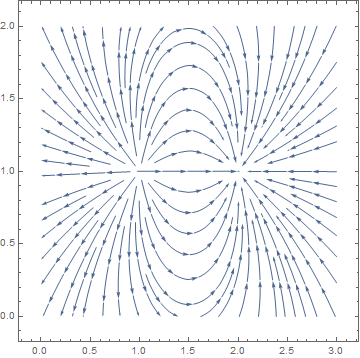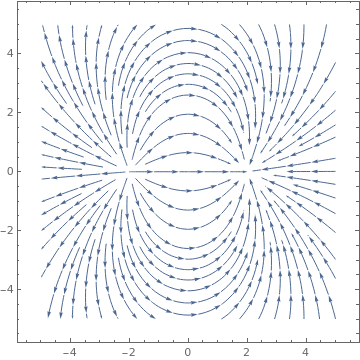Another version of the same plot: charge[q_, {x0_, y0_, z0_}][x_, y_, z_] := q/((x - x0)^2 + (y - y0)^2 + (z - z0)^2)^(3/2) {x - x0, y - y0, z - z0}; projector[{x_, y_, z_}] := {x, y} VectorPlot[ projector[ charge[1, {0, 4, 0}][x, y, 0] + charge[-1, {0, -4, 0}][x, y, 0]], {x, -10, 10}, {y, -10, 10}]; StreamPlot[ projector[ charge[1, {-2, 0, 0}][x, y, 0] + charge[-1, {2, 0, 0}][x, y, 0]], {x, -5, 5}, {y, -5, 5}] Electric field potential of a dipole. Mathematica code

First, we define the Coulomb fields at the origin using "Ec" in the code below
Ec[x_,y_] := {x/(x^2 + y^2)^(3/2), y/(x^2 + y^2)^(3/2)};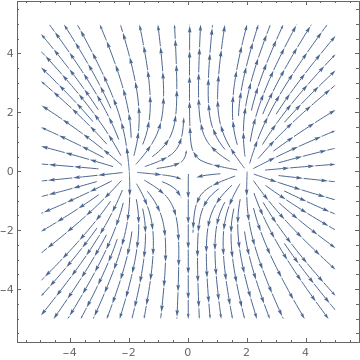A completely different vector field is obtained when we add two equal charges: Ec[x_, y_] := {x/(x^2 + y^2)^(3/2), y/(x^2 + y^2)^(3/2)}; StreamPlot[Ec[x + 2, y] + Ec[x - 2, y], {x, -5, 5}, {y, -5, 5}] Electric field potential of two equal charges. Mathematica code

We can visualize vector fields in 3-dimensional space.    ■
Example 2: The vector [-2, 8, 5, 0] is a linear combination of the vectors [3, 1, -2, 2], [1, 0, 3, -1], and [4, -2, 1 0], because it is the sum of scalar multiples of the three vectors:
$2\,[3,\, 1,\, -2,\,2] + 4\,[1,\,0,\,3,\,-1] -3\,[4,\,-2,\, 1,\, 0] = [-2,\,8,\, 5,\, 0] . \qquad ■$

■

Both a vector and a matrix can be multiplied by a scalar; with the operation being *. Matrices and vectors can be added or subtracted only when their dimensions are the same.

Let S be a subset of a vector space V.

(1) S is a linearly independent subset of V if and only if no vector in S can be expressed as a linear combination of the other vectors in S.
(2) S is a linearly dependent subset of V if and only if some vector v in S can be expressed as a linear combination of the other vectors in S.

Theorem 1: A nonempty set $$S = \{ {\bf v}_1 , \ {\bf v}_2 , \ \ldots , \ {\bf v}_r \}$$ of nonzero vectors in a vector space V is linearly independent if and only if the only coefficients satisfying the vector equation
$k_1 {\bf v}_1 + k_2 {\bf v}_2 + \cdots + k_r {\bf v}_r = {\bf 0}$
are $$k_1 =0, \ k_2 =0, \ \ldots , \ k_r =0 .$$

Theorem 2: A nonempty set $$S = \{ {\bf v}_1 , \ {\bf v}_2 , \ \ldots , \ {\bf v}_r \}$$ of r nonzero vectors in a vector space V is linearly independent if and only if the matrix of the column-vectors from S has rank r.

Let $$S = \{ {\bf v}_1 , \ {\bf v}_2 , \ \ldots , \ {\bf v}_n \}$$ be a set of vectors in a finite-dimensional vector space V. Then S is called basis for V if:

• S spans V;
• S is linearly independent.   ▣

Vector products

Mathematica has three multiplication commands for vectors: the dot (or inner) and outer products (for arbitrary vectors), and the cross product (for three dimensional vectors).

For three dimensional vectors $${\bf a} = a_1 \,{\bf i} + a_2 \,{\bf j} + a_3 \,{\bf k} = \left[ a_1 , a_2 , a_3 \right]$$ and $${\bf b} = b_1 \,{\bf i} + b_2 \,{\bf j} + b_3 \,{\bf k} = \left[ b_1 , b_2 , b_3 \right]$$ , it is possible to define special multiplication, called the cross-product:

${\bf a} \times {\bf b} = \det \left[ \begin{array}{ccc} {\bf i} & {\bf j} & {\bf k} \\ a_1 & a_2 & a_3 \\ b_1 & b_2 & b_3 \end{array} \right] = {\bf i} \left( a_2 b_3 - b_2 a_3 \right) - {\bf j} \left( a_1 b_3 - b_1 a_3 \right) + {\bf k} \left( a_1 b_2 - a_2 b_1 \right) .$

The cross product can be done on two vectors. It is important to note that the cross product is an operation that is only functional in three dimensions. The operation can be computed using the Cross[vector 1, vector 2] operation or by generating a cross product operator between two vectors by pressing [Esc] cross [Esc]. ([Esc] refers to the escape button)

Cross[{1,2,7}, {3,4,5}]
{-18,16,-2}

The dot product of two vectors of the same size $${\bf x} = \left[ x_1 , x_2 , \ldots , x_n \right]$$ and $${\bf y} = \left[ y_1 , y_2 , \ldots , y_n \right]$$ (regardless of whether they are columns or rows because Mathematica does not distinguish rows from columns) is the number, denoted either by $${\bf x} \cdot {\bf y}$$ or $$\left\langle {\bf x} , {\bf y} \right\rangle ,$$

$\left\langle {\bf x} , {\bf y} \right\rangle = {\bf x} \cdot {\bf y} = x_1 y_1 + x_2 y_2 + \cdots + x_n y_n ,$
when entries are real, or
$\left\langle {\bf x} , {\bf y} \right\rangle = {\bf x} \cdot {\bf y} = \overline{x_1} y_1 + \overline{x_2} y_2 + \cdots + \overline{x_n} y_n ,$

when entries are complex. Here $$\overline{\bf x} = \overline{a + {\bf j}\, b} = a - {\bf j}\,b$$ is a complex conjugate of a complex number x = a + jb.

The dot product of any two vectors of the same dimension can be done with the dot operation given as Dot[vector 1, vector 2] or with use of a period “. “ .

{1,2,3}.{2,4,6}
28
Dot[{1,2,3},{3,2,1} ]
10
With Euclidean norm ‖·‖2, the dot product formula
${\bf x} \cdot {\bf y} = \| {\bf x} \|_2 \, \| {\bf y} \|_2 \, \cos \theta ,$
defines θ, the angle between two vectors. The dot product was first introduced by the American physicist and mathematician Josiah Willard Gibbs (1839--1903) in the 1880s. ■

An outer product is the tensor product of two coordinate vectors $${\bf u} = \left[ u_1 , u_2 , \ldots , u_m \right]$$ and $${\bf v} = \left[ v_1 , v_2 , \ldots , v_n \right] ,$$ denoted $${\bf u} \otimes {\bf v} ,$$ is an m-by-n matrix W of rank 1 such that its coordinates satisfy $$w_{i,j} = u_i v_j .$$ The outer product $${\bf u} \otimes {\bf v} ,$$ is equivalent to a matrix multiplication $${\bf u} \, {\bf v}^{\ast} ,$$ (or $${\bf u} \, {\bf v}^{\mathrm T} ,$$ if vectors are real) provided that u is represented as a column $$m \times 1$$ vector, and v as a column $$n \times 1$$ vector. Here $${\bf v}^{\ast} = \overline{{\bf v}^{\mathrm T}} .$$

Example 3: Taking, for instance, m = 4 and n = 3, we have
${\bf u} \otimes {\bf v} = {\bf u} \, {\bf v}^{\mathrm T} = \begin{bmatrix} u_1 \\ u_2 \\ u_3 \\ u_4 \end{bmatrix} \begin{bmatrix} v_1 & v_2 & v_3 \end{bmatrix} = \begin{bmatrix} u_1 v_1 & u_1 v_2 & u_1 v_3 \\ u_2 v_1 & u_2 v_2 & u_2 v_3 \\ u_3 v_1 & u_3 v_2 & u_3 v_3 \\ u_4 v_1 & u_4 v_2 & u_4 v_3 \end{bmatrix} .$
If we take two vectors $${\bf u} = [1, 2, 3, 4]$$ and $${\bf v} = [-1, 0, 2] ,$$ then their outer product is
${\bf u} \otimes {\bf v} = {\bf u} \, {\bf v}^{\mathrm T} = \begin{bmatrix} -1 &0&2 \\ -2&0&4 \\ -3&0&6 \\ -4&0&8 \end{bmatrix} ,$
{{1}, {2}, {3}, {4}}.{{-1, 0, 2}}
MatrixRank[%]
Out= 1
which is rank 1 matrix. ■

An inner product of two vectors of the same size, usually denoted by $$\left\langle {\bf x} , {\bf y} \right\rangle ,$$ is a generalization of the dot product if it satisfies the following properties:

• $$\left\langle {\bf v}+{\bf u} , {\bf w} \right\rangle = \left\langle {\bf v} , {\bf w} \right\rangle + \left\langle {\bf u} , {\bf w} \right\rangle .$$
• $$\left\langle {\bf v} , \alpha {\bf u} \right\rangle = \alpha \left\langle {\bf v} , {\bf u} \right\rangle$$ for any scalar α.
• $$\left\langle {\bf v} , {\bf u} \right\rangle = \overline{\left\langle {\bf u} , {\bf v} \right\rangle} ,$$ where overline means complex conjugate.
• $$\left\langle {\bf v} , {\bf v} \right\rangle \ge 0 ,$$ and equal if and only if $${\bf v} = {\bf 0} .$$

The fourth condition in the list above is known as the positive-definite condition. A vector space together with the inner product is called an inner product space. Every inner product space is a metric space. The metric or norm is given by

$\| {\bf u} \| = \sqrt{\left\langle {\bf u} , {\bf u} \right\rangle} .$
The nonzero vectors u and v of the same size are orthogonal (or perpendicular) when their inner product is zero: $$\left\langle {\bf u} , {\bf v} \right\rangle = 0 .$$ We abbreviate it as $${\bf u} \perp {\bf v} .$$ If A is an n × n positive definite matrix and u and v are n-vectors, then we can define the weighted Euclidean inner product
$\left\langle {\bf u} , {\bf v} \right\rangle = {\bf A} {\bf u} \cdot {\bf v} = {\bf u} \cdot {\bf A}^{\ast} {\bf v} \qquad\mbox{and} \qquad {\bf u} \cdot {\bf A} {\bf v} = {\bf A}^{\ast} {\bf u} \cdot {\bf v} .$
In particular, if w1, w2, ... , wn are positive real numbers, which are called weights, and if u = ( u1, u2, ... , un) and v = ( v1, v2, ... , vn) are vectors in ℝn, then the formula
$\left\langle {\bf u} , {\bf v} \right\rangle = w_1 u_1 v_1 + w_2 u_2 v_2 + \cdots + w_n u_n v_n$
defines an inner product on $$\mathbb{R}^n ,$$ that is called the weighted Euclidean inner product with weights w1, w2, ... , wn.
Example 4: The Euclidean inner product and the weighted Euclidean inner product (when $$\left\langle {\bf u} , {\bf v} \right\rangle = \sum_{k=1}^n a_k u_k v_k ,$$ for some positive numbers $$a_k , \ (k=1,2,\ldots , n$$ ) are special cases of a general class of inner products on $$\mathbb{R}^n$$ called matrix inner product. Let A be an invertible n-by-n matrix. Then the formula
$\left\langle {\bf u} , {\bf v} \right\rangle = {\bf A} {\bf u} \cdot {\bf A} {\bf v} = {\bf v}^{\mathrm T} {\bf A}^{\mathrm T} {\bf A} {\bf u}$
defines an inner product generated by A.

Example 5: In the set of integrable functions on an interval [a,b], we can define the inner product of two functions f and g as
$\left\langle f , g \right\rangle = \int_a^b \overline{f} (x)\, g(x) \, {\text d}x \qquad\mbox{or} \qquad \left\langle f , g \right\rangle = \int_a^b f(x)\,\overline{g} (x) \, {\text d}x .$
Then the norm $$\| f \|$$ (also called the 2-norm or 𝔏² norm) becomes the square root of
$\| f \|^2 = \left\langle f , f \right\rangle = \int_a^b \left\vert f(x) \right\vert^2 \, {\text d}x .$
In particular, the 2-norm of the function $$f(x) = 5x^2 +2x -1$$ on the interval [0,1] is
$\| 2 x^2 +2x -1 \| = \sqrt{\int_0^1 \left( 5x^2 +2x -1 \right)^2 {\text d}x } = \sqrt{7} .$
Example 6: Consider a set of polynomials of degree n. If
${\bf p} = p(x) = p_0 + p_1 x + p_2 x^2 + \cdots + p_n x^n \quad\mbox{and} \quad {\bf q} = q(x) = q_0 + q_1 x + q_2 x^2 + \cdots + q_n x^n$
are two polynomials, and if $$x_0 , x_1 , \ldots , x_n$$ are distinct real numbers (called sample points), then the formula
$\left\langle {\bf p} , {\bf q} \right\rangle = p(x_0 ) q(x_0 ) + p_1 (x_1 )q(x_1 ) + \cdots + p(x_n ) q(x_n )$
defines an inner product, which is called the evaluation inner product at $$x_0 , x_1 , \ldots , x_n .$$

The invention of Cartesian coordinates in 1649 by René Descartes (Latinized name: Cartesius) revolutionized mathematics by providing the first systematic link between Euclidean geometry and algebra.

Vector norms

In order to define how close two vectors are, and in order to define the convergence of sequences of vectors, we can use the notion of a norm. We will heavily use the following notation for nonnegative real numbers:
$\mathbb{R}_{+} = \left\{ x \in \mathbb{R} \, : \, x\ge 0 \right\} .$
Besides real numbers, we will also use the field of complex numbers that consists of all ordered pairs (𝑎, b) = 𝑎 + jb with appropriate addition and multiplication operations. The unit vector in positive vertical direction is denoted by j, so that j² = −1. Also recall that if z = 𝑎 + jb ∈ ℂ is a complex number, with real numbers 𝑎, b ∈ ℝ, then its complex conjugate is $$\overline{z} = a - {\bf j}b ,$$ and $$|z| = |\overline{z}| = \sqrt{a^2 + b^2}$$ is the modulus of z.
Let V be a vector space over either the field of real numbers ℝ or complex numbers ℂ. A norm on V is a function from a real or complex vector space V to the nonnegative real numbers ℝ+ that satisfies the following conditions:
• Positivity:     ‖v‖ ≥ 0,     ‖v‖ = 0 iff v = 0.
• Homogeneity:     ‖kv‖ = |k| ‖v‖ for arbitrary scalar k.
• Triangle inequality:     ‖v + u‖ ≤ ‖u‖ + ‖v‖.
A vector space together with a norm ‖·‖ is called a normed vector space.
We mention four of many norms:
• For every x = [x1, x2, … , xn] ∈ V, we have the 1-norm:
$\| {\bf x}\|_1 = \sum_{k=1}^n | x_k | = |x_1 | + |x_2 | + \cdots + |x_n |.$
It is also called the Taxicab norm or Manhattan norm.
• The Euclidean norm or ℓ²-norm is
$\| {\bf x}\|_2 = \left( \sum_{k=1}^n x_k^2 \right)^{1/2} = \left( x_1^2 + x_2^2 + \cdots + x_n^2 \right)^{1/2} .$
• The Chebyshev norm or sup-norm ‖v, is defined such that
$\| {\bf x}\|_{\infty} = \max_{1 \le k \le n} \left\{ | x_k | \right\} .$
• The ℓp-norm (for p≥1)
$\| {\bf x}\|_p = \left( \sum_{k=1}^n x_k^p \right)^{1/p} = \left( x_1^p + x_2^p + \cdots + x_n^p \right)^{1/p} .$
Theorem 3: The following inequalities hold for all x ∈ ℂn or x ∈ ℝn:
• $$\displaystyle \| {\bf x} \|_{\infty} \le \| {\bf x} \|_{1} \le n\,\| {\bf x} \|_{\infty} ,$$
• $$\displaystyle \| {\bf x} \|_{\infty} \le \| {\bf x} \|_{2} \le \sqrt{n}\,\| {\bf x} \|_{\infty} ,$$
• $$\displaystyle \| {\bf x} \|_{2} \le \| {\bf x} \|_{1} \le \sqrt{n}\,\| {\bf x} \|_{2} .$$
In given any (real or complex) vector space V, two norms ‖ ‖a and ‖ ‖b are equivalent if and only if (iff) there exists some positive constants c1 and c2 such that
$\| {\bf x}\|_a \le c_1 \| {\bf x}\|_b \qquad \mbox{and} \qquad \| {\bf x}\|_b \le c_2 \| {\bf x}\|_a \qquad \mbox{for all } \quad {\bf x} \in V.$

Theorem 4: If V is any real or complex vector space of finite dimension, then any two norms on V are equivalent.

With dot product, we can assign a length of a vector, which is also called the Euclidean norm or 2-norm:

$\| {\bf x} \|_2 = \sqrt{ {\bf x}\cdot {\bf x}} = \sqrt{x_1^2 + x_2^2 + \cdots + x_n^2} .$
An inner product space is a vector space with an additional structure called an inner product. So every inner product space inherits the Euclidean norm and becomes a metric space. In linear algebra, functional analysis, and related areas of mathematics, a norm is a function that assigns a strictly positive length or size to each vector in a vector space—save for the zero vector, which is assigned a length of zero. On an n-dimensional complex space $$\mathbb{C}^n ,$$ the most common norm is
$\| {\bf z} \| = \sqrt{ {\bf z}\cdot {\bf z}} = \sqrt{\overline{z_1} \,z_1 + \overline{z_2}\,z_2 + \cdots + \overline{z_n}\,z_n} = \sqrt{|z_1|^2 + |z_2 |^2 + \cdots + |z_n |^2} .$
A unit vector u is a vector whose length equals one: $${\bf u} \cdot {\bf u} =1 .$$ We say that two vectors x and y are perpendicular if their inner product is zero.

For any norm, the Cauchy--Bunyakovsky--Schwarz (or simply CBS) inequality holds:

\begin{equation} \label{EqVector.1} | {\bf x} \cdot {\bf y} | \le \| {\bf x} \| \, \| {\bf y} \| . \end{equation}
For p ≥ 1, we define q as
$\frac{1}{p} + \frac{1}{q} = 1 .$
Then the CBS inequality can be generalized as
\begin{equation} \label{EqVector.2} | {\bf x} \cdot {\bf y} | \le \| {\bf x} \|_p \, \| {\bf y} \|_q . \end{equation}
Eq.\eqref{EqVector.2} is known as "Hölder's inequality.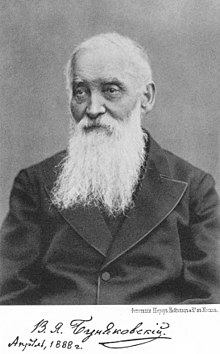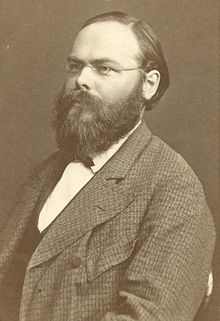Augustin-Louis Cauchy    Viktor Yakovlevich Bunyakovsky    Hermann Amandus Schwarz
The inequality for sums was published by Augustin-Louis Cauchy (1789--1857) in 1821, while the corresponding inequality for integrals was first proved by Viktor Yakovlevich Bunyakovsky (1804--1889) in 1859. The modern proof (which is actually a repetition of the Bunyakovsky's one) of the integral inequality was given by Hermann Amandus Schwarz (1843--1921) in 1888.   ■